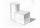# Surface area + expression of a variable from the formula - math problems

#### Number of problems found: 172

• Cube and sphereCube with the surface area 150 cm2 is described sphere. What is sphere surface?
• Lateral surface areaThe ratio of the area of the base of the rotary cone to its lateral surface area is 3: 5. Calculate the surface and volume of the cone, if its height v = 4 cm.
• Area to volumeIf the surface area of a cube is 486, find its volume.
• Spherical cap 4What is the surface area of a spherical cap, the base diameter 20 m, height 2.5 m? Calculate using formula.
• Surface areaThe volume of a cone is 1000 cm3 and the content area of the axis cut is 100 cm2. Calculate the surface area of the cone.
• Cone - from volume surface areaThe volume of the rotating cone is 1,018.87 dm3, its height is 120 cm. What is the surface area of the cone?
• Cube wallsFind the volume and the surface area of the cube if the area of one of its walls is 40 cm2.
• AirplaneAviator sees part of the earth's surface with an area of 200,000 square kilometers. How high he flies?
• Surface area of cylinderDetermine the lateral surface of the rotary cylinder which is circumscribed cube with edge length 5 cm.
• Area of a cubeCalculate the surface area of a cube if its volume is equal to 729 cubic meters.
• Cube wallsFind the volume and surface area of the cube if the area of one wall is 40cm2.
• PilotHow high is the airplane's pilot to see 0.001 of Earth's surface?
• Surface of the cylinderCalculate the surface of the cylinder for which the shell area is Spl = 20 cm2 and the height v = 3.5 cm
• The cylinderThe cylinder has a surface area of 300 square meters, while the height of the cylinder is 12 m. Calculate the volume of this cylinder.
• Volume and surfaceCalculate the volume and surface area of the cylinder when the cylinder height and base diameter is in a ratio of 3:4 and the area of the cylinder jacket is 24 dm2.
• TerezaThe cube has an area of base 256 mm2. Calculate the edge length, volume, and area of its surface.
• Edge cFind the edge c of cuboid if an edge a = 20 mm, b = 30 mm and surface area S = 8000 mm2.
• Cube surface and volumeFind the surface of the cube with a volume of 27 dm3.
• Angle of deviationThe surface of the rotating cone is 30 cm2 (with circle base), its surface area is 20 cm2. Calculate the deviation of the side of this cone from the plane of the base.
• The volumeThe volume of the sphere is 1 m3, what is its surface?

Do you have an interesting mathematical word problem that you can't solve it? Submit a math problem, and we can try to solve it.

We will send a solution to your e-mail address. Solved examples are also published here. Please enter the e-mail correctly and check whether you don't have a full mailbox.

Please do not submit problems from current active competitions such as Mathematical Olympiad, correspondence seminars etc...